## Example Questions

### Example Question #21 : Geometry

The formula to find the radius of the largest circle that can fit in an equilateral triangle is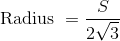, where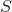is the length of any one side of the triange.

What is the largest diameter of a circle that can fit inside an equilateral triangle with a perimeter ofcm?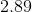cm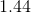cm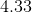cm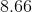cmcm

Explanation:

The diameter is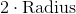To solve for the largest diameter multiply each side by 2.

The resulting formula for diamenter is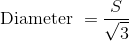.

Substitute in 5  for S and solve. Diameter =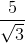= 2.89 cm

### Example Question #24 : Geometry

Quantity A: The diameter of a circle with area of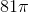Quantity B: The diameter of a circle with circumference of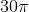Which of the following is true?

Quantity A is larger.

Both quantities are equal.

Quantity B is larger.

The relationship of the quantities cannot be determined.

Quantity B is larger.

Explanation:

Consider each quantity separately.

Quantity A

Recall that the area of a circle is defined as:We know that the area is. Therefore,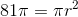Divide both sides by: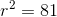Therefore,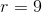. Since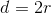, we know: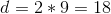Quantity B

This is very easy. Recall that: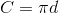Therefore, if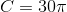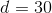. Therefore, Quantity B is larger.

### Example Question #25 : Geometry

Quantity A: The diameter of a circle with area of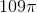Quantity B: The diameter of a circle with circumference of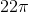Which of the following is true?

The two quantities are equal.

The relationship between the quantities cannot be determined.

Quantity B is larger.

Quantity A is larger.

Quantity B is larger.

Explanation:

Consider each quantity separately.

Quantity A

Recall that the area of a circle is defined as:We know that the area is. Therefore,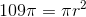Divide both sides by: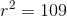Therefore,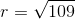.  Since, we know: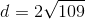Quantity B

This is very easy.  Recall that:Therefore, if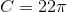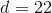.

Now, since your calculator will not have a square root button on it, we need to estimate for Quantity A. We know that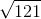is. Therefore,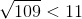.  This means that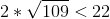. Therefore, Quantity B is larger.

### Example Question #26 : Geometry

A circle with an area ofis divided into sectors with areas in a ratio of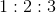. What is the area of the largest sector?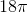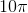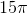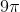Explanation:

From the ratio given, it may be easier to write it such that the terms sum up to. This can be taken by dividing each term by the sum of the terms: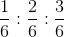or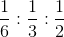The largest sector thus has an area equal to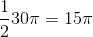### Example Question #27 : Geometry

A rectangle is inscribed inside of a circle such that every corner touches the edge of the circle. If the area of the rectangle is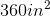and the perimeter of the rectangle is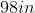, what is the area of the circle in inches squared?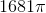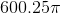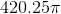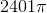Explanation:

To find the area of the circle, it is important to know either its diameter or radius. For the geometry described in this problem, this is the same as the diagonal of the rectangle.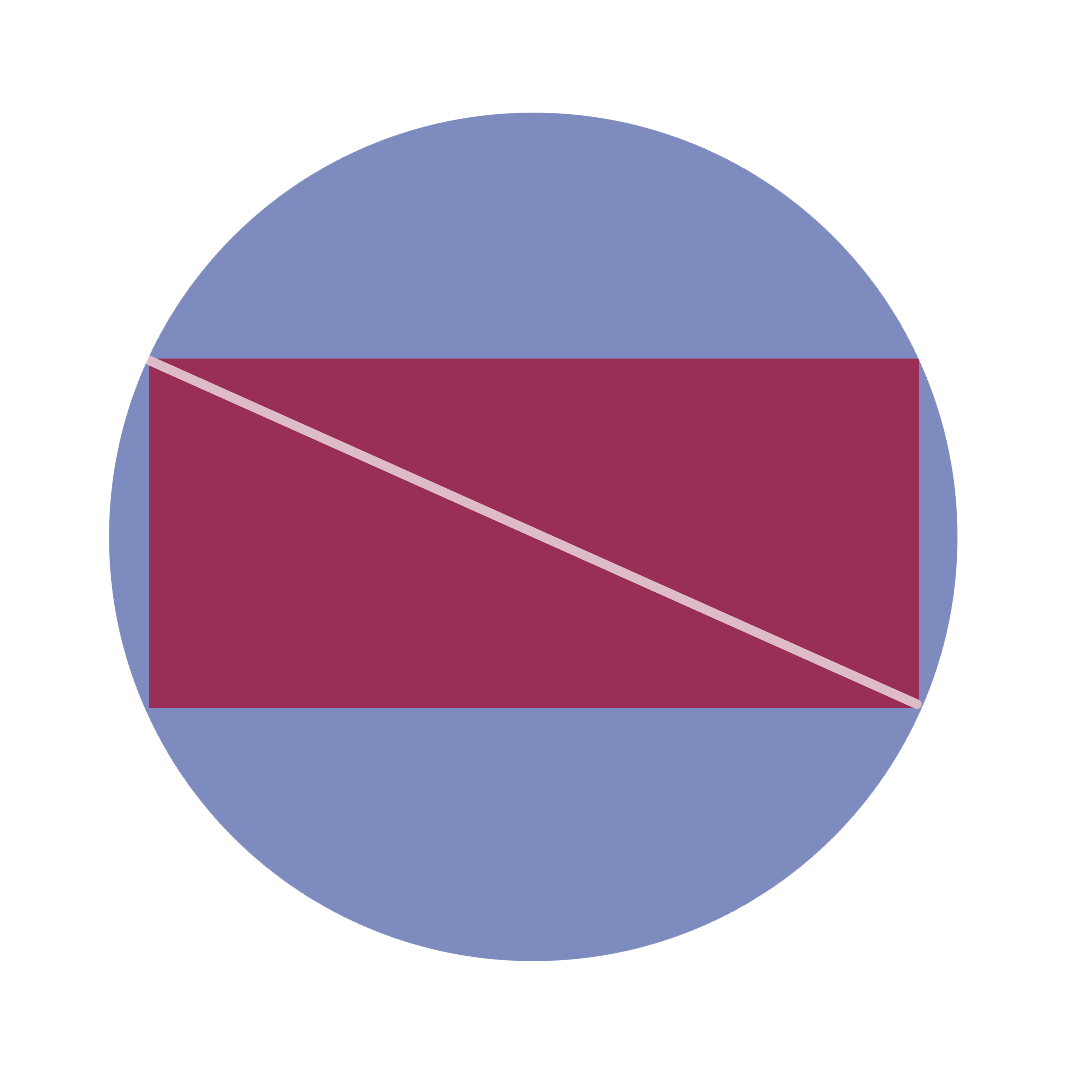However, to find the diagonal of the rectangle, the sides must first be known. They can be found, since the perimeter and area are given: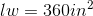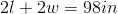This system of equation can be solved by substitution: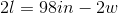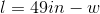Followed by: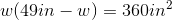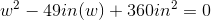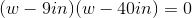Note that this gives two possible values for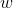,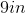or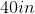, though the one selected is irrelevant; the other value will be the value for.

Knowing these two values, the diagonal can be found; it is the hypotenuse of a right triangle formed by these two lengths: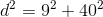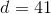Since the diagonal is also the diameter, the area of the circle is given by: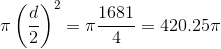Tired of practice problems?

Try live online GRE prep today.